# Simple Thermal Heat Transfer Finite Element Analysis in Excel 2007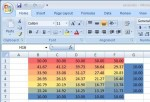While some free finite element analysis software is available, the engineer or student can learn a lot about how FEA works by constructing a simple model from scratch in a commonly available spreadsheet program such as Excel.  The technique presented here can simulate a two dimensional flat plate of uniform thickness.  Each boundary element can be an arbitrary fixed-temperature or insulated.  The resultant temperature of each active element is iteratively calculated by simply averaging the temperatures of the adjacent cells.

1. Open a new workbook. We need to set Excel to allow circular references and do iterative calculations. Start by clicking on the Office Button, then “Excel Options”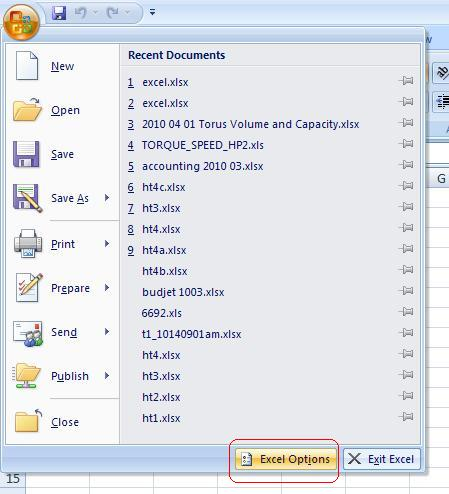2. Click the “Formulas” tab and check the “Enable iterative calculation” check box. Leave the default values of 100 iterations and 0.001 change for now. We’ll also leave “Workbook Calculation to “Automatic” but you may want to change it to “Manual” later if your model becomes larger and more complex. When you’re done, click “OK”.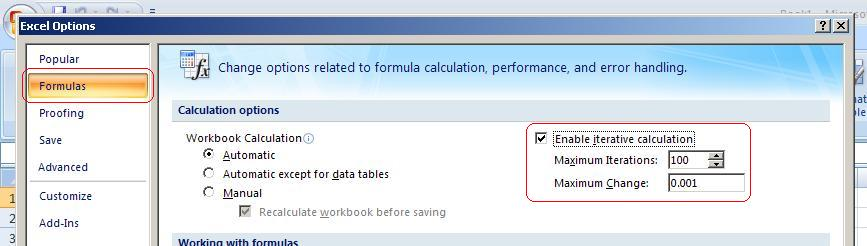3. Decide the parameters of your model. In this example, we’ll simulate a plate that is five inches by five inches. Each spreadsheet cell will represent a 1″x1″ element. We’ll start with a uniform, fixed-temperature boundary condition on all four sides at 10 degrees.

4. Set up the boundary temperatures. In rows 1 and 7, enter a “10” in columns B through F. In columns A and G, enter a 10 in rows 2 through 6. The plate will be represented by the cells surrounded by these boundaries. While you’re at it, select the entire area from A1 to G7 and format it as “Number” with the default two decimal places.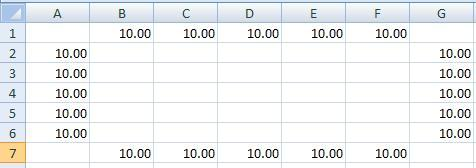5. Set up the first element formula. In cell B2, type “=AVERAGE(B1,C2,B3,A2)”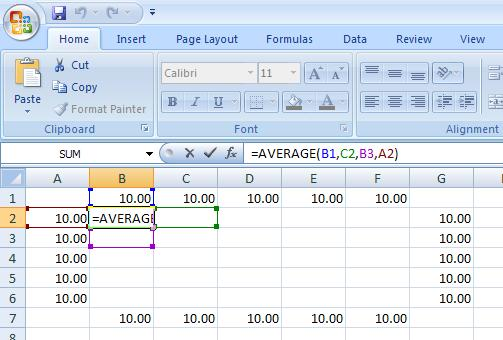6. Copy this formula to fill the area inside the boundaries (the range between B2 and F6). Everything should calculate out to 10. Next, we’ll start to make it more interesting.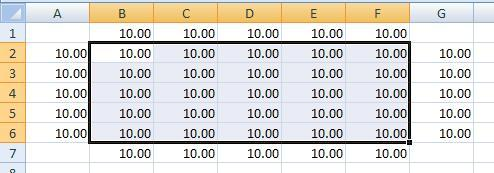7. Change the row 1 boundary temperatures to 50. As soon as you change the first sell, you’ll start to see the numbers change as the elements recalculate. Next we’ll add some color.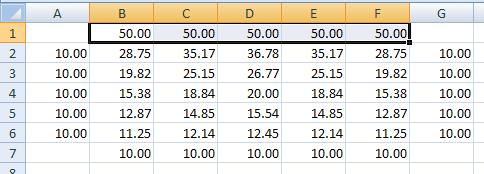8. Select the entire area from A1 to G7 and then on the “Home” tab click “Conditional Formatting”, “Color Scales”, “Red – Yellow – Blue Color scale”. Now the temperature distribution should be easy to visualize.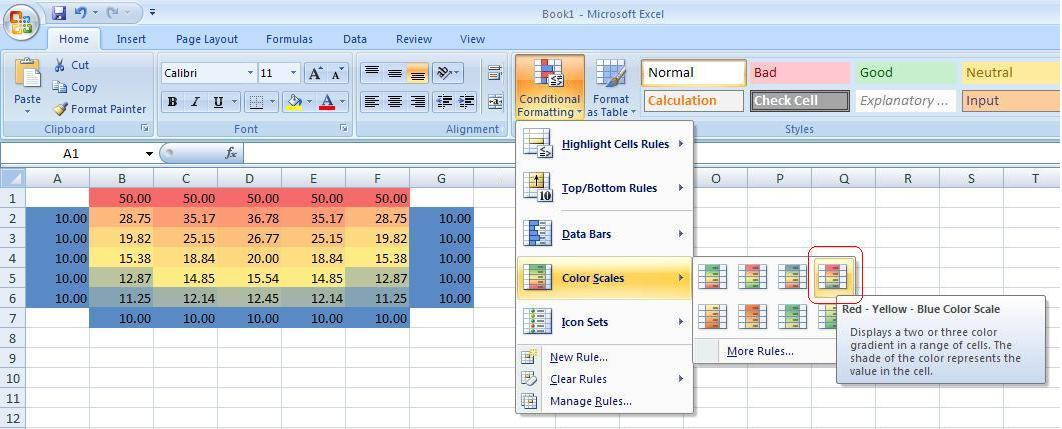9. Change the left side boundary to an insulated condition. Click on cell B2 and edit the formula to “=AVERAGE(B1,C2,B3)”, removing the reference to the cell to its left (A2). This cell is now insulated on the left side.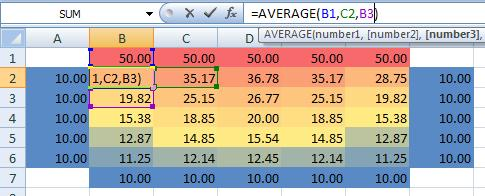10. Copy this cell to cells B2 through B6 to insulate the entire side. You can delete the now-unused fixed 10 degree values on column A to prevent confusion. Notice how the temperature distribution has changed.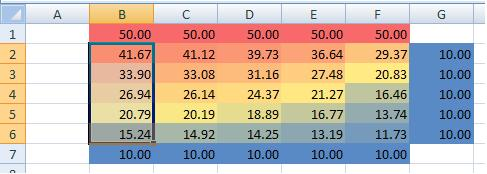11. Now feel free to experiment with changing the boundary temperature values and adding and removing boundary conditions. To review the status of any cell, select that cell and then click on the text of the formula in the formula bar to place the cursor there. The cell references in the formula will be color coded to correspond with colored borders around each adjacent cell that is in the formula.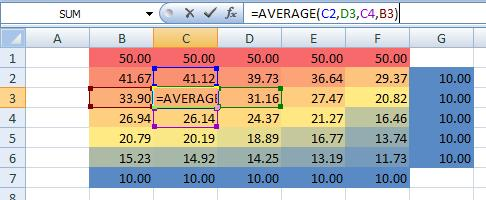Note that the thermal conductivity value of the material does not need to be considered.  Likewise, the units of temperature are not important as long as the same units are used throughout.

The default 100 iterations may not be enough to reach stable values.  You can start a manual recalculation by hitting F9.

Other conditions such as convection or radiation at the boundaries or heat generation such as an electric heating element can be added to the model, but the affected element formulas become more complex.

## 2 thoughts on “Simple Thermal Heat Transfer Finite Element Analysis in Excel 2007”

1. I teach a physics course and would like to use this as a laboratory exercise. Can you tell me the source of this exercise? Can you suggest any other possible activities, like a hole in the center for example.

By the way, this is great, really gives the fundamental concepts of FEA, thank you

2.Tom Scarince on said:

Thanks for your comment, Doug. The concept was taken and simplified from some long forgotten heat transfer textbook. I’ve actually used this technique several times over the years to help solve real engineering problems.

It is helpful when you know the heat distibution at the boundaries from empirical data. You can then reconstruct the internal heat distribution.

The delta-T between two adjacent cells tells you about the heat flux between them.

You can model whatever shape you like. For instance, deletesome cells in the center to make a “hole”.

By the way, I now realize that step 9 is not needed. You can leave all four adjacent cells in the formula and when you delete the “insulated” cells in step ten the blank cells are ignored.

Finally, you can view a walk-through video of the above tutorial here:
http://youtu.be/Qo39bkAPTTo

Please let me know if you have any questions.